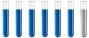## Electron-transfer reaction

Chemistry and homework help forum.

Organic Chemistry, Analytical Chemistry, Biochemistry, Physical Chemistry, Computational Chemistry, Theoretical Chemistry, High School Chemistry, Colledge Chemistry and University Chemistry Forum.

Share your chemistry ideas, discuss chemical problems, ask for help with scientific chemistry questions, inspire others by your chemistry vision!

Please feel free to start a scientific chemistry discussion here!

Discuss chemistry homework problems with experts!

Ask for help with chemical questions and help others with your chemistry knowledge!

Moderators: expert, ChenBeier, Xen

Dhamnekar Winod
Sr. Staff MemberPosts: 192
Joined: Sat Nov 21, 2020 10:14 am
Location: Mumbai[Bombay],Maharashtra State,India

### Electron-transfer reaction

1)What current will be required to produce 1.0 g of Na in 10.0 min. by electrolysis of molten NaCl?

2) What quantity of electricity will provide 0.624 mol of electrons?

3)If the current is 3.5 A, how many Coulombs per second are passing through the cell?

4) How many minutes will be required for a current 3.5 A , to produce 6.02 × 10⁴ C?
Last edited by Dhamnekar Winod on Tue Jul 27, 2021 10:00 pm, edited 1 time in total.
Any science consists of the following process. 1) See 2)Hear 3)Smell,if needed 4)Taste, if needed 5)Think 6)Understand 7)Inference 8)Take decision [Believe or disbelieve, useful or useless, true or false, cause or effect, any other criteria]
ChenBeier
Distinguished MemberPosts: 873
Joined: Wed Sep 27, 2017 7:25 am
Location: Berlin, Germany

### Re: Electron-transfer reaction

The basic is the law of faraday

m = I * t * c
c = electrochemical equivalent =M/ (z *F)

m= mass, I = current, t= time, M = molar mass, z = amount of transferred electrons, F = Faraday constant 96485 As,
Dhamnekar Winod
Sr. Staff MemberPosts: 192
Joined: Sat Nov 21, 2020 10:14 am
Location: Mumbai[Bombay],Maharashtra State,India

### Re: Electron-transfer reaction

1)$$\frac{1 g}{22.99 g/mol Na} \times \frac {1 mol e^-}{1mol Na} \times \frac{96485.3399 C}{1 mol e^-} \frac{1}{10 min \times 60 s/min}= 7.0 C = 7.0 A$$ current will be required to produce 1 g of Na in 10.0 minutes by the electrolysis of molten NaCL

2)$$0.624 mol e^- \times \frac{96485.3399 C}{1 mol e^- }= 6.02 \times 10^4 C$$ elecectricity will provide 0.624 mol e⁻.

3) If the current is 3.5 A, 3.5 Coulombs per second are passing through the cell.

4)$$\frac{6.02 \times 10^4 C}{3.5 C/s} \times \frac {1 min}{60 s} = 2.90 \times 10^2 min$$ will be required for a current of 3.5 A to produce $$6.02 \times 10^4 C$$ electricity
Any science consists of the following process. 1) See 2)Hear 3)Smell,if needed 4)Taste, if needed 5)Think 6)Understand 7)Inference 8)Take decision [Believe or disbelieve, useful or useless, true or false, cause or effect, any other criteria]
ChenBeier
Distinguished MemberPosts: 873
Joined: Wed Sep 27, 2017 7:25 am
Location: Berlin, Germany

Looks ok.